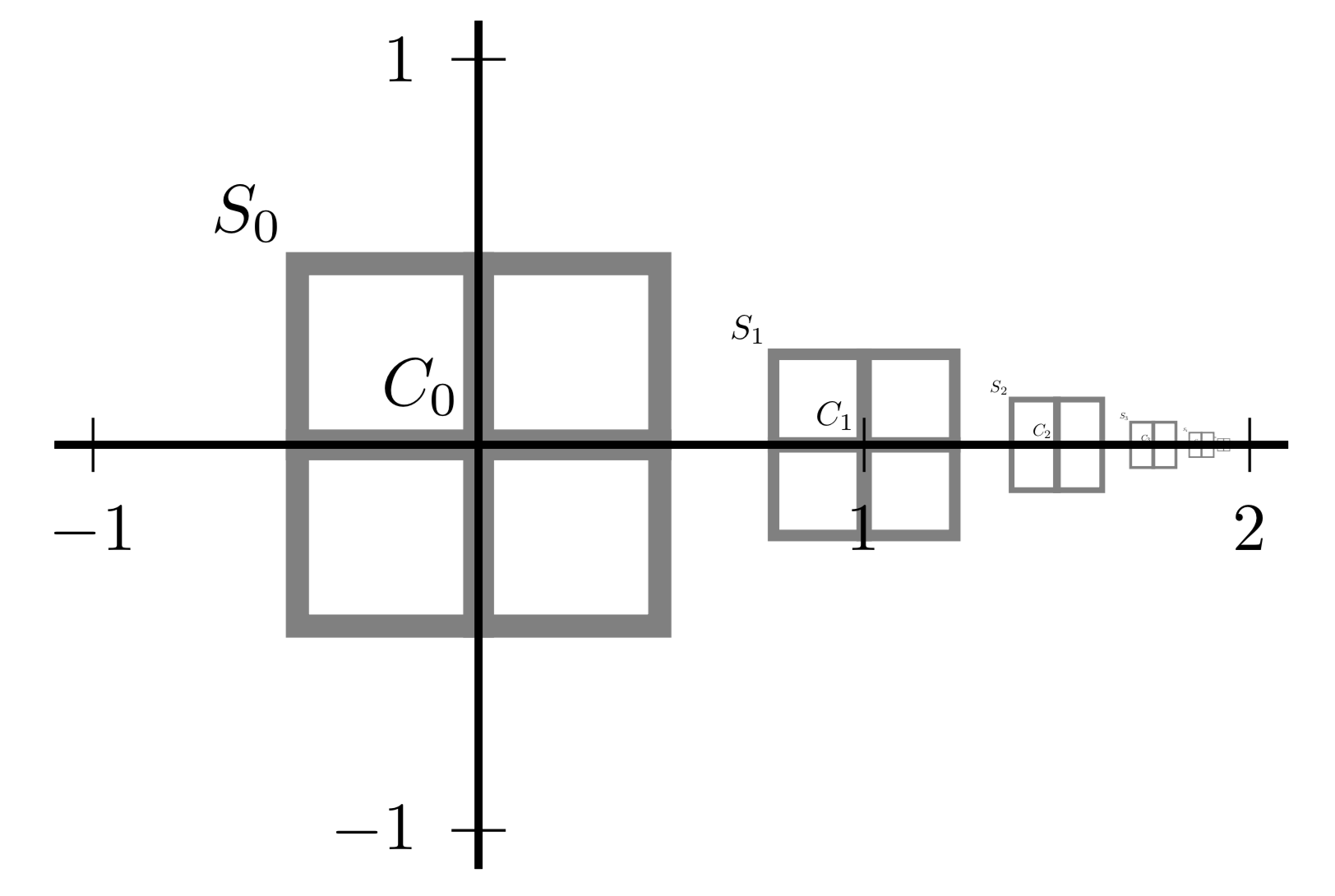# Tim Sullivan### Weak and strong modes

Han Cheng Lie and I have just uploaded a revised preprint of our paper, “Equivalence of weak and strong modes of measures on topological vector spaces”, to the arXiv. This addresses a natural question in the theory of modes (or maximum a posteriori estimators, in the case of posterior measure for a Bayesian inverse problem) in an infinite-dimensional space $$X$$. Such modes can be defined either strongly (a la Dashti et al. (2013), via a global maximisation) or weakly (a la Helin and Burger (2015), via a dense subspace $$E \subset X$$). The question is, when are strong and weak modes equivalent? The answer turns out to be rather subtle: under reasonable uniformity conditions, the two kinds of modes are indeed equivalent, but finite-dimensional counterexamples exist when the uniformity conditions fail.

Abstract. A strong mode of a probability measure on a normed space $$X$$ can be defined as a point $$u \in X$$ such that the mass of the ball centred at $$u$$ uniformly dominates the mass of all other balls in the small-radius limit. Helin and Burger weakened this definition by considering only pairwise comparisons with balls whose centres differ by vectors in a dense, proper linear subspace $$E$$ of $$X$$, and posed the question of when these two types of modes coincide. We show that, in a more general setting of metrisable vector spaces equipped with non-atomic measures that are finite on bounded sets, the density of $$E$$ and a uniformity condition suffice for the equivalence of these two types of modes. We accomplish this by introducing a new, intermediate type of mode. We also show that these modes can be inequivalent if the uniformity condition fails. Our results shed light on the relationships between among various notions of maximum a posteriori estimator in non-parametric Bayesian inference.

Published on Monday 9 July 2018 at 08:00 UTC #preprint #inverse-problems #modes #map-estimators #lie